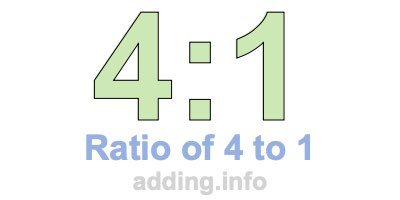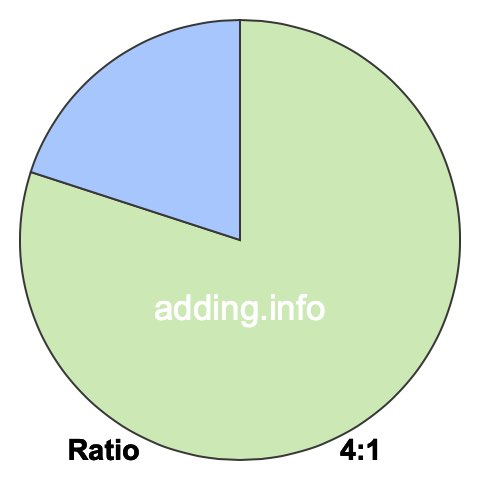Ratio of 4 to 1A ratio of 4 to 1 can be written as 4 to 1, 4:1, or 4/1. Furthermore, 4 and 1 can be the quantity or measurement of anything, such as students, fruit, weights, heights, speed and so on.

A ratio of 4 to 1 simply means that for every 4 of something, there are 1 of something else, with a total of 5.

In the box below, you can enter a number in either box and we will calculate the other number to keep the ratio of 4 to 1. In other words, we will calculate the corresponding equivalent ratio of 4:1 for you.

:

To calculate the left side of the ratio, our calculator multiplies the right side by 4 and then divides the product by 1. To calculate the right side of the ratio, our calculator multiplies the left side by 1 and then divides the product by 4. Answers are rounded to two decimals if necessary.

Below is a list of equivalent ratios of 4:1 that we created using our 4:1 ratio calculator above.

4:1

8:2

12:3

16:4

20:5

24:6

28:7

32:8

36:9

40:10

44:11

48:12

We have also created a pie chart for you to put 4:1 ratio in perspective. To do so, we divided a pie into 5 pieces and then colored 4 pieces green and 1 pieces blue. The pie will look exactly the same using any equivalent ratio of 4:1.The green part of the pie covers 80% and the blue part covers 20%. To calculate the percentages, we divided each side of the ratio by its total and then multiplied by 100, like this:

(4 / 5)×100 = 80
(1 / 5)×100 = 20

In fractional terms, 4/1 of the pie above is colored in green. This means that the ratio of 4 to 1 or 4:1 can also be expressed as a fraction, where the 4 to the left of the colon is the numerator and the 1 to the right of the colon is the denominator:

4:1 = 4/1

Ratio is often used when defining rectangles in width:height format. Width and height can be feet, inches, centimeters, or any other kind of length. With a ratio of 4 to 1, the width is 4 and the height is 1. Below is a picture of what a rectangle with a ratio of 4:1 looks like.Since the simplest form of the fraction 4/1 is 4/1, the simplest form of the ratio 4:1 is also 4:1.

Ratio Calculator
Need information about another ratio? Here you can submit another ratio for us to explain.

:

Ratio of 4 to 2
Go to the next ratio on our list that we have detailed information about.